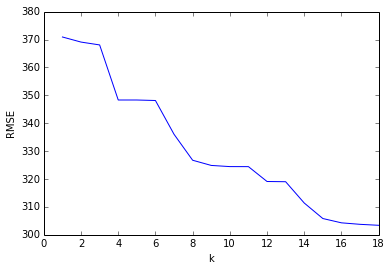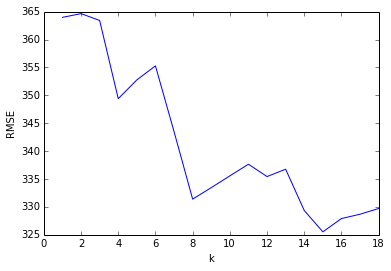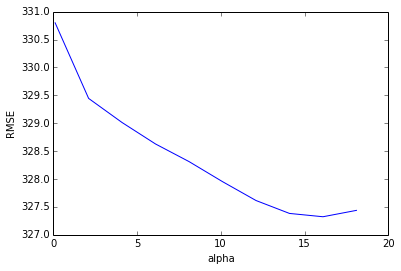Chapter 6: Feature Selection¶

In :
from __future__ import division
import pandas as pd
import numpy as np
import scipy as sp
import matplotlib.pyplot as plt
from sklearn.linear_model import LinearRegression
from sklearn.linear_model import Ridge
from sklearn.linear_model import Lasso
from sklearn.feature_selection import SelectKBest
from sklearn.feature_selection import f_regression
from sklearn.metrics import mean_squared_error
from sklearn.cross_validation import KFold
%matplotlib inline
In :
hitters_df.dropna(inplace=True)
Out:
AtBat Hits HmRun Runs RBI Walks Years CAtBat CHits CHmRun CRuns CRBI CWalks League Division PutOuts Assists Errors Salary NewLeague
1 315 81 7 24 38 39 14 3449 835 69 321 414 375 N W 632 43 10 475.0 N
2 479 130 18 66 72 76 3 1624 457 63 224 266 263 A W 880 82 14 480.0 A
3 496 141 20 65 78 37 11 5628 1575 225 828 838 354 N E 200 11 3 500.0 N
4 321 87 10 39 42 30 2 396 101 12 48 46 33 N E 805 40 4 91.5 N
5 594 169 4 74 51 35 11 4408 1133 19 501 336 194 A W 282 421 25 750.0 A

5 rows × 20 columns

Objective is to predict the hitter's salary given other variables. Because this is a regression problem, we first need to convert the non-numeric input variables to factors.

In :
# Converting non-numeric input variables to factors.
hitters_df["League"] = pd.factorize(hitters_df["League"])
hitters_df["Division"] = pd.factorize(hitters_df["Division"])
hitters_df["NewLeague"] = pd.factorize(hitters_df["NewLeague"])
Out:
AtBat Hits HmRun Runs RBI Walks Years CAtBat CHits CHmRun CRuns CRBI CWalks League Division PutOuts Assists Errors Salary NewLeague
1 315 81 7 24 38 39 14 3449 835 69 321 414 375 0 0 632 43 10 475.0 0
2 479 130 18 66 72 76 3 1624 457 63 224 266 263 1 0 880 82 14 480.0 1
3 496 141 20 65 78 37 11 5628 1575 225 828 838 354 0 1 200 11 3 500.0 0
4 321 87 10 39 42 30 2 396 101 12 48 46 33 0 1 805 40 4 91.5 0
5 594 169 4 74 51 35 11 4408 1133 19 501 336 194 1 0 282 421 25 750.0 1

5 rows × 20 columns

In :
# construct a baseline regressor with all features
collist = [col for col in hitters_df.columns if col != "Salary"]
X = hitters_df[collist]
y = hitters_df["Salary"]
reg = LinearRegression()
reg.fit(X, y)
ypred = reg.predict(X)
np.sqrt(mean_squared_error(ypred, y))
Out:
303.34447253531613

Best Subset Regression¶

R's leap package allows for multiple ways to find the best K features for a model - by exhaustive search and forward (greedy) search. Scikit-Learn offers the SelectKBest method to find the best features, presumably using a greedy search.

In :
mses = []
nfeatures = range(1, len(collist))
for nfeature in nfeatures:
# compute MSE for different values of k (top features)
selector = SelectKBest(f_regression, k=nfeature)
selector.fit(X, y)
selected = selector.get_support()
feats = [col for (col,sel) in zip(collist, selected) if sel]
reg = LinearRegression()
X_r = hitters_df[feats]
reg.fit(X_r, y)
ypred = reg.predict(X_r)
mses.append(np.sqrt(mean_squared_error(ypred, y)))
plt.plot(nfeatures, mses)
plt.xlabel("k")
plt.ylabel("RMSE")
Out:Model Selection by Cross-Validation¶

The RMSE falls as the number of features increase - this is expected because we are computing the RMSE off the training set (overfitting). We will now use 10-fold cross validation on each model to calculate a cross-validation MSE which will give us a better idea of the best feature size to use for the problem.

In :
cv_errors = []
kfold = KFold(len(hitters_df), n_folds=10)
nfeatures = range(1, len(collist))
for nfeature in nfeatures:
# build model with varying number of features
selector = SelectKBest(f_regression, k=nfeature)
selector.fit(X, y)
selected = selector.get_support()
feats = [col for (col,sel) in zip(collist, selected) if sel]
X_r = hitters_df[feats].values
y = hitters_df["Salary"].values
rmses = []
for train, test in kfold:
# each model is cross validated 10 times
Xtrain, ytrain, Xtest, ytest = X_r[train], y[train], X_r[test], y[test]
reg = LinearRegression()
reg.fit(Xtrain, ytrain)
ypred = reg.predict(Xtest)
rmses.append(np.sqrt(mean_squared_error(ypred, ytest)))
cv_errors.append(np.mean(rmses))
plt.plot(nfeatures, cv_errors)
plt.xlabel("k")
plt.ylabel("RMSE")
Out:
<matplotlib.text.Text at 0x4c84710>Ridge Regression and the Lasso¶

These two methods improve the model by using all features but shrinking the coefficients. Ridge regression uses the L2-norm and Lasso regression uses the L1-norm. Here we use cross validation to compute the RMSE for a baseline model, a model regularized by Ridge regression and one regularized using Lasso.

In :
def cross_validate(X, y, nfolds, reg_name):
rmses = []
kfold = KFold(X.shape, n_folds=nfolds)
for train, test in kfold:
Xtrain, ytrain, Xtest, ytest = X[train], y[train], X[test], y[test]
reg = None
if reg_name == "ridge":
reg = Ridge()
elif reg_name == "lasso":
reg = Lasso()
else:
reg = LinearRegression()
reg.fit(Xtrain, ytrain)
ypred = reg.predict(Xtest)
rmses.append(np.sqrt(mean_squared_error(ytest, ypred)))
return np.mean(rmses)

collist = [col for col in hitters_df.columns if col != "Salary"]
X = hitters_df[collist].values
y = hitters_df["Salary"].values
rmse_baseline = cross_validate(X, y, 10, "baseline")
rmse_ridge = cross_validate(X, y, 10, "ridge")
rmse_lasso = cross_validate(X, y, 10, "lasso")
(rmse_baseline, rmse_ridge, rmse_lasso)
Out:
(331.00425667935832, 330.72572506106593, 330.00460161612779)

Finally, we attempt to find an optimum value of alpha for the Lasso regressor using cross-validation.

In :
cv_errors = []
alphas = [0.1 * alpha for alpha in range(1, 200, 20)]
kfold = KFold(X.shape, n_folds=10)
for alpha in alphas:
rmses = []
for train, test in kfold:
Xtrain, ytrain, Xtest, ytest = X[train], y[train], X[test], y[test]
reg = Lasso(alpha=alpha)
reg.fit(Xtrain, ytrain)
ypred = reg.predict(Xtest)
rmses.append(np.sqrt(mean_squared_error(ytest, ypred)))
cv_errors.append(np.mean(rmses))
plt.plot(alphas, cv_errors)
plt.xlabel("alpha")
plt.ylabel("RMSE")
Out:
<matplotlib.text.Text at 0x4ef3590>